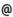## "Babes-Bolyai" University of Cluj-Napoca Faculty of Mathematics and Computer Science

 Differential equations
 Code Semes-ter Hours: C+S+L Credits Type Section MME0001 2 2+2+0 6 compulsory Matematică MME0001 2 2+1+1 5 compulsory Matematică informatică MME0001 2 2+2+0 6 compulsory Matematici aplicate
 Teaching Staff in Charge
 Prof. PETRUSEL Adrian Olimpiu, Ph.D., petruselmath.ubbcluj.ro
 Prof. PRECUP Radu, Ph.D., r.precupmath.ubbcluj.ro
 Assoc.Prof. BEGE Antal, Ph.D., begemath.ubbcluj.ro
 Aims Introduction to the basic problems of ordinary differential equations (Cauchy problem, boundary value problems) as well as the discussion of some mathematical models governed by differential equations.
 Content 1. Mathematical models governed by differential equations: Mathematical modelling, examples. 2. Differential and integral equations completely solvable. 3. The Cauchy problem: Exiastence, uniqueness and data dependence 4. N-order linear differential equations 5. First order linear systems of differential equations 6. Dynamical aspects in the theory of differential equations: dynamical systems generated by differential equations, flow anf phase portrait. 7. Stability theory: Stability for linear differentail equations.
 References 1. I. A. RUS, Ecuatii diferentiale, ecuatii integrale si sisteme dinamice, Transilvania Press, Cluj-Napoca, 1996. 2. I. A. RUS, P. PAVEL, Ecuatii diferentiale, Ed. Didactica, Bucuresti, 1982. 3. V. BARBU, Ecuatii diferentiale, Ed. Junimea, Iasi, 1985. 4. D. V. IONESCU, Ecuatii diferentiale si integrale, Ed. Didactica, Bucuresti, 1972. 5. L. PERKO, Differential Equations and Dynamical Systems, Springer-Verlag, New York, 2001. 6. G. Morosanu, Ecuatii diferentiale. Aplicatii, Editura Academiei, 1990. 7. GH. MICULA, P. PAVEL, Ecuatii diferentiale si integrale prin exercitii si probleme, Editura Dacia, 1989. 8. J. C. ROBINSON, An introduction to ordinary differential equations. Cambridge University Press, Cambridge, 2004.
 Assessment Control paper (20 % of the final mark), written examination (50%) and oral examination (30%).